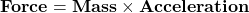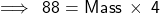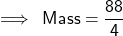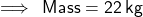## given a force of 88n and an acceleration of 4m/s^2 what is the mass

Question

given a force of 88n and an acceleration of 4m/s^2 what is the mass

in progress 0
6 months 2021-07-16T09:35:20+00:00 1 Answers 16 views 0

1. ### Given,

Force = 88 N

Acceleration = 4 m/s²

### Weknowthat,★ Substituting the values in the above formula,we get: